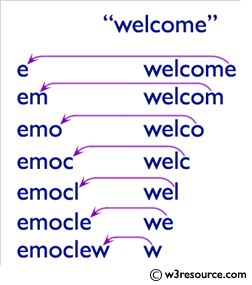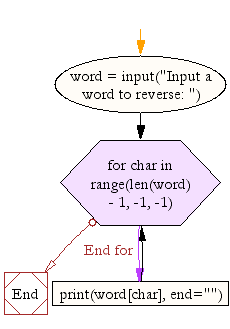﻿ Python Exercise: Reverse a word - w3resource# Python Exercise: Reverse a word

## Python Conditional: Exercise-5 with Solution

Write a Python program that accepts a word from the user and reverse it.

Pictorial Presentation:Sample Solution:

Python Code:

``````word = input("Input a word to reverse: ")

for char in range(len(word) - 1, -1, -1):
print(word[char], end="")
print("\n")
```
```

Sample Output:

```Input a word to reverse: ecruoser3w
w3resource
```

Flowchart:## Visualize Python code execution:

The following tool visualize what the computer is doing step-by-step as it executes the said program:

Python Code Editor:

Have another way to solve this solution? Contribute your code (and comments) through Disqus.

What is the difficulty level of this exercise?

Test your Python skills with w3resource's quiz

﻿

## Python: Tips of the Day

Returns a list with n elements removed from the beginning

Example:

```def tips_take(itr, n = 1):
return itr[:n]
print(tips_take([1, 2, 3], 5))
print(tips_take([1, 2, 3], 0))
```

Output:

```[1, 2, 3]
[]
```